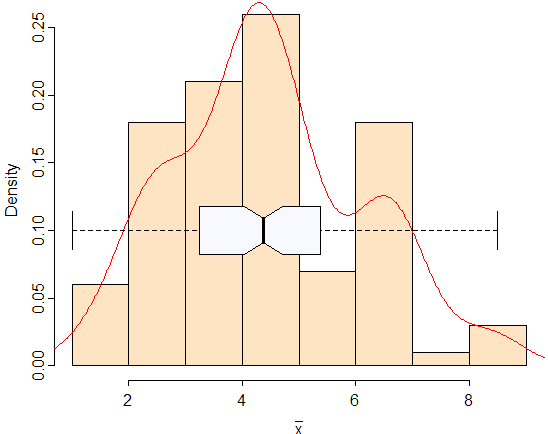Animated Statistics Using R (to be moved to AniWiki!)
Resampling... Empirical distribution... Computer-intensive... Free of complicated mathematical derivations...
Ideas of Bootstrapping

Before I start, I'd like to recommend the book An Introduction to the Bootstrap by Efron and Tibshirani for those who want to know further about bootstrapping, because what I will introduce here is rather superficial.

The two critical points for bootstrapping are: (1) data generating mechanism; (2) plug-in principle. The first tells us how to re-generate data from a sample, while the latter point tells us how to make estimations. The idea of bootstrapping is based on the method of resampling to a large degree. In the real world, we only have one sample, say, n sample points x1, x2, ..., xn, then the problems we must face (when making inferences) are:

• How to guarantee the population distribution which we have assumed is correct?
• How to derive the expression of the point estimate or confidence interval of a parameter if the population distribution is tooooooo complicated?
• Or how can we obtain the distribution of a statistic when the population distribution is complicated?

We have always been deriving mathematical formulae... for this statistic... for that statistic... under perfect but unwarranted assumptions...

Why not re-generate some samples (resample the original sample with replacement) and re-compute the values of our statistic of interest? Then we can get a series of estimations of a certain parameter and in a result, we are able to make inferences based on these numbers using the plug-in principle, e.g. we may compute the standard error of a parameter by compute the corresponding standard error of that series of numbers (please do note the factual computation is not exactly so; read the references to learn the details), and estimate the quantiles of a statistic just by computing the corresponding quantiles of that series of numbers, etc. If you are confused by my description here, just keep on to the next section: an example might help you.

Implementation by Computers

Simple applications of bootstrapping with the help of computers can be extremely easy in R. Actually all we have to do is specify an argument replace = TRUE of the function sample(). I think everybody with a little knowledge of statistics know the SRSWOR. Here is a simple example:

```> (x = 1:10)
  1  2  3  4  5  6  7  8  9 10
> sample(x, 5, replace = FALSE) # no duplicated sample points
 7 3 4 1 5
> sample(x, 5, replace = TRUE) # note there are two 8s
 2 9 4 8 8
```

If we repeat the resampling procedure for many times, we may, at the same time, compute the value of the statistic of interest for many times with different samples. Using "plug-in" principle, we can approximately obtain the distribution of that statistic. For example, now we have a sample which consists of four numbers {1, 3, 4, 10}, and we want to examine the distribution of the sample mean:

```> x = c(1, 3, 4, 10)
> (x.mean = replicate(100, mean(sample(x, replace = T))))
  6.25  4.50  5.25  2.50  5.00  2.25  1.75 10.00  2.50  2.75
  5.25  4.50  6.25  6.25  5.25  6.50  3.50  4.75  1.75  5.25
  3.25  4.75  3.25  2.25  2.75  3.75  5.50  6.50  3.25  4.00
  5.50  4.25  6.75  4.75  2.25  4.75  6.75  4.75  2.25  2.75
  2.75  3.75  5.25  3.00  6.75  1.75  3.50  3.75  6.75  1.50
  2.25  7.00  6.00  5.25  4.00  7.75  2.25  4.50  5.50  2.50
  2.50  3.75  4.50  4.00  6.50  4.50  3.75  6.75  4.25  5.50
  4.50  6.25  8.50  4.50  4.75  2.25  2.50  2.50  3.50  5.50
  6.75  4.25  6.00  4.25  4.75  3.00  1.75  5.00  3.50  4.75
  3.50  6.00  4.00  6.25  3.75  8.50  3.00  6.25  4.25  6.25
```

I used the function replicate() to repeat the resampling: every time we got a "new" sample, we just computed a sample mean. After 100 replications, we got 100 mean values. And we can easily check the distribution of these numbers by histograms or other approaches. Below is a histogram with a density curve (use R functions hist() and density()):Figure 1 The distribution of the sample mean by bootstrapping.

Note that we can accordingly compute the estimations of the variance or quantiles of the sample mean using the above result. (e.g. sd(x.mean), quantile(x.mean), etc.)

Demonstration

Let's generate some random numbers from U(0, 1) and check the distribution of the sample mean as the bootstrapping goes on. Blue points represent the original sample, and the red points mean these points are what we get by resampling (because they are resampled with replacement, the times of points being picked out are denoted by sunflower plots -- the number of "leaves" means times of being selected). Then we compute the sample mean based on these red points and plot its density accordingly.